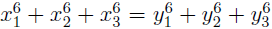# On two Diophantine equations 2A6 + B6 = 2C6 ± D3

Susil Kumar Jena
Notes on Number Theory and Discrete Mathematics, ISSN 1310-5132
Volume 20, 2014, Number 2, Pages 29—34

## Details

### Authors and affiliations

Susil Kumar JenaDepartment of Electronics and Telecommunication Engineering
KIIT University
Bhubaneswar 751024, Odisha, India

### Abstract

We give parametric solutions, and thus show that the two Diophantine equations 2A6 + B6 = 2C6 ± D3 have infinitely many nontrivial and primitive solutions in positive integers (A, B, C, D).

### Keywords

• Diophantine equation
• Diophantine equation 2A6 + B6 = 2C6 + D3
• Diophantine equation 2A6 + B6 = 2C6D3
• Equal sums of higher powers

• 11D41
• 11D72

### References

1. Bremner, A. A geometric approach to equal sums of sixth powers, Proc. London Math. Soc., Vol. 43, 1981, 544–581.
2. Brudno, S. On generating infinitely many solutions of the Diophantine equation A6 + B6 + C6 = D6 + E6 + F6, Math. Comp., Vol. 24, 1970, 453–454.
3. Brudno, S. Triples of sixth powers with equal sums, Math. Comp., Vol. 30, 1976, 646–648.
4. Choudhry, A. On equal sums of sixth powers, Indian J. Pure Appl. Math., Vol. 25, 1994, 837–841.
5. Choudhry, A. On equal sums of sixth powers, Rocky Mountain J. Math., Vol. 30, 2000, 843–848.
6. Delorme, J.-J. On the Diophantine equation, Math. Comput., Vol. 59, 1992, 703–715.

## Cite this paper

Kumar Jena, S. (2014). On two Diophantine equations 2A6 + B6 = 2C6 ± D3. Notes on Number Theory and Discrete Mathematics, 20(2), 29-34.